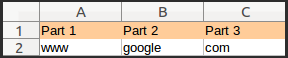# String Processing in LibreOffice Calc Macro with Examples

## 3 min

In this tutorial, we will show how to do various common string processing using macro in LibreOffice. We will use LibreOffice Calc cells to use the strings for this tutorial. These processings are essential for any macro development.

Lets put 3 strings like below in a LibreOffice calc sheet and join them.## Joining Strings in Calc

In basic, to join two strings/texts, use “&” operator. We will use this to join three columns of strings and put the results in another column.

```Sub String_join()
part1 = ThisComponent.Sheets(0).getCellByPosition(0,1)
part2 = ThisComponent.Sheets(0).getCellByPosition(1,1)
part3 = ThisComponent.Sheets(0).getCellByPosition(2,1)

result_cell = ThisComponent.Sheets(0).getCellByPosition(3,1)
result_cell.String = part1.String & "." & part2.String & "." & part3.String
End Sub
```

Lets put some more strings in Column A as below in LibreOffice. We will use these three strings for rest of the processings in this tutorial.

## Left

Left function returns the number of characters from a string from left side. The number of character can be specified in the argument of the function. Put a string in cell A1 – “www.google.com” and run the function on the A1 cell as below. This function will return sub-string “www”.

```Sub left_demo()
part1 = ThisComponent.Sheets(0).getCellByPosition(0,0)
result = ThisComponent.Sheets(0).getCellByPosition(2,0)
result.String = left(part1.String,3)
End Sub
```

## Right

Just like left function above, right returns the given no of character from right side. With the same example, in this case, below function would return “com”.

```Sub right_demo()
part1 = ThisComponent.Sheets(0).getCellByPosition(0,0)
result = ThisComponent.Sheets(0).getCellByPosition(2,0)
result.String = right(part1.String,3)
End Sub
```

## Mid

Mid function returns any size of sub-string from a given string. The arguments of this function is Mid(string, start position, length of the sub-string). To have Mid function in works, put some strings in a column in LibreOffice calc and run the Mid function. In this below example, the strings from A columns is converted to sub-string which is in column B. The sub-string is starting from character 4 of the string and length is 6 characters.

```Sub mid_demo()
part1 = ThisComponent.Sheets(0).getCellByPosition(0,1)
result = ThisComponent.Sheets(0).getCellByPosition(1,1)
result.String = mid(part1.String,5,6)

part2 = ThisComponent.Sheets(0).getCellByPosition(0,2)
result = ThisComponent.Sheets(0).getCellByPosition(1,2)
result.String= mid(part2.String,5,6)

part3 = ThisComponent.Sheets(0).getCellByPosition(0,3)
result = ThisComponent.Sheets(0).getCellByPosition(1,3)
result.String = mid(part3.String,5,6)
End Sub
```

## Trim

Trim function is used to remove leading and trailing spaces from a string.
The function can be used like this: `Trim(your_string_here)`

## Len

Len function returns the number of character present i.e. the length of a string.
Usage: `Len(string)`

## Instr

Instr function is used to search a string within a string. If match found, Instr returns the starting position number of the searched string and if not found, it returns 0.

```Sub instr_demo()
part1 = ThisComponent.Sheets(0).getCellByPosition(0,1)
result = ThisComponent.Sheets(0).getCellByPosition(1,1)
result.Value = instr(part1.String,"oogl")

part2 = ThisComponent.Sheets(0).getCellByPosition(0,2)
result = ThisComponent.Sheets(0).getCellByPosition(1,2)
result.Value = instr(part2.String,"dd")

part3 = ThisComponent.Sheets(0).getCellByPosition(0,3)
result = ThisComponent.Sheets(0).getCellByPosition(1,3)
result.Value = instr(part3.String,"untu")
End Sub
```

In this tutorial, basic string processing is covered which can be used in LibreOffice Macro.

SEE ALSO:   Do you want to change the look of LibreOffice default toolbar?

## Looking for Something Else?

We bring the latest tech, software news and stuff that matters. Stay in touch via Telegram, Twitter, YouTube, and Facebook and never miss an update!## Like it? Share with your friends!

Subscribe
Notify ofThis site uses Akismet to reduce spam. Learn how your comment data is processed.